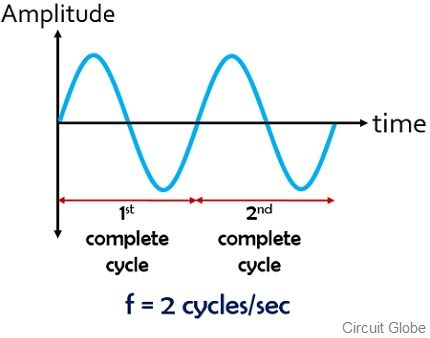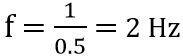# Difference Between Period and Frequency

Time period and frequency are the two major parameters associated with the wave. Period and frequency are exactly opposite of each other. The crucial difference between period and frequency is that period is the duration in which a complete wave cycle is achieved. As against frequency is the number of complete cycles of a wave occurring in a specific amount of time.

Basically, a wave is generated from back and forth movement which is nothing but the oscillation of the object. We know oscillation is associated with motion. Thus the oscillating body has some force and energy. Therefore oscillation is said to be a periodic motion, that gets repeated at regular time durations.

Generally, a wave is measured on the basis of wave parameters which are nothing but amplitude, period, frequency, phase, etc. The term period and frequency are the two most confusing terms related to wave. So, here we will discuss the difference between the two in various aspects.

## Comparison Chart

Basis for ComparisonPeriodFrequency
BasicIt defines the duration in which a wave cycle is completed in unit time.The number of overall complete cycles appearing in specific time.
DenotedTf
Specified asseconds/cyclecycles/second
NatureTime quantityRate Quantity
UnitSecondsHertz

### Definition of Period

The period is defined as the smallest time interval required by a wave to complete a wave cycle. It is denoted by ‘T’. Consider this figure:As we can see that here x-axis is the time axis while the y-axis is representing the amplitude. Thus the minimum time required by the wave after which it repeats the cycle is represented as T. This is nothing but the time period of the wave.

To have a better understanding consider that a nail is beaten by a hammer. Suppose in 1 second the nail is hammered 2 times. Thus the time utilized for 1 complete hammering process in a single second will be given as:Thus, the period will be 0.5 seconds for each cycle. Hence is specified in terms of second/cycle.

### Definition of Frequency

The number of complete wave repetitions occurring in a unit time is referred as frequency. And ‘f’ is the denotion used for it. Consider the waveform representation given below:Again here also the x-axis represents the time parameter in seconds. And y-axis shows the magnitude of the wave. So, the number of complete cycles that occurred in a specific time interval say per second is denoted by f i.e., the frequency of the wave.

Again consider the previously taken example of beating a nail with a hammer. As we have considered previously that in 1 second, the hammering is done twice. So, we can say that in the overall duration of 1 second the complete hammering process is occurring two times. Thus frequency, in this case, will be 2. Conversely, we can say that if a complete cycle of process is taking 0.5 seconds to happen then in a second the process is occurring twice. Thus is given as:Thus is specified in terms of cycle/second.

## Key Differences Between Period and Frequency

1. The period of a wave often referred as the time period is the measure of time required by a wave for completing one cycle. However, frequency is the overall number of cycles occurring in unit time.
2. The period of a wave is time quantity as its measurement is associated with time duration. While frequency is a rate quantity because it measures the total number of cycles appearing.
3. The period of a wave in a generalized manner is given as seconds/cycle. But the frequency of a wave is given as cycles/ second.
4. The lower case letter, ‘f’ is the representation used for frequency. While the denotation used for the time period is the upper case letter ‘T’.
5. As the period is time quantity thus its unit is seconds. Whereas frequency is a rate quantity, therefore, is measured in hertz.

### Conclusion

The parameters period and frequency are inverse of each other. Hence is given as:So, due to the inverse relationship between the two, it can be concluded that the higher the frequency, the lower will be the time period and vice-versa.